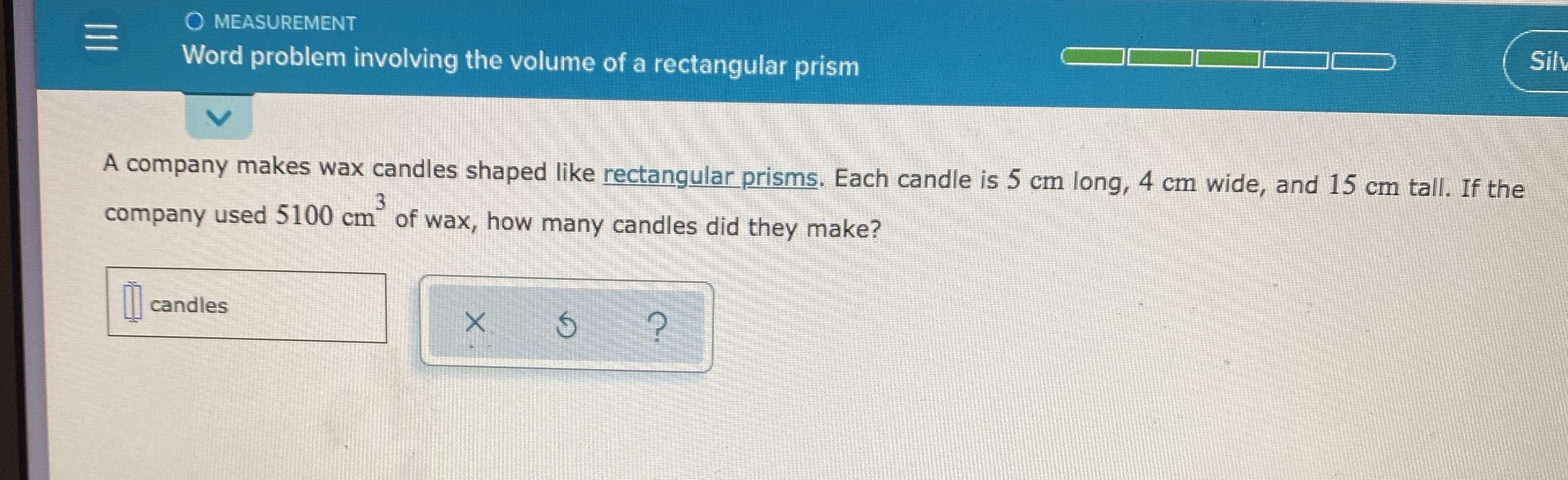¿Todavía tienes preguntas de matemáticas?

Pregunte a nuestros tutores expertos
Geometry
QuestionA company makes wax candies shaped like rectangular prisms.Each candies is $$5$$   $$cm$$ long and $$15$$    $$cm$$ tall.If the company used  $$5100$$   $$cm^{2}$$ of wax,how many candies did they make?

$$17$$